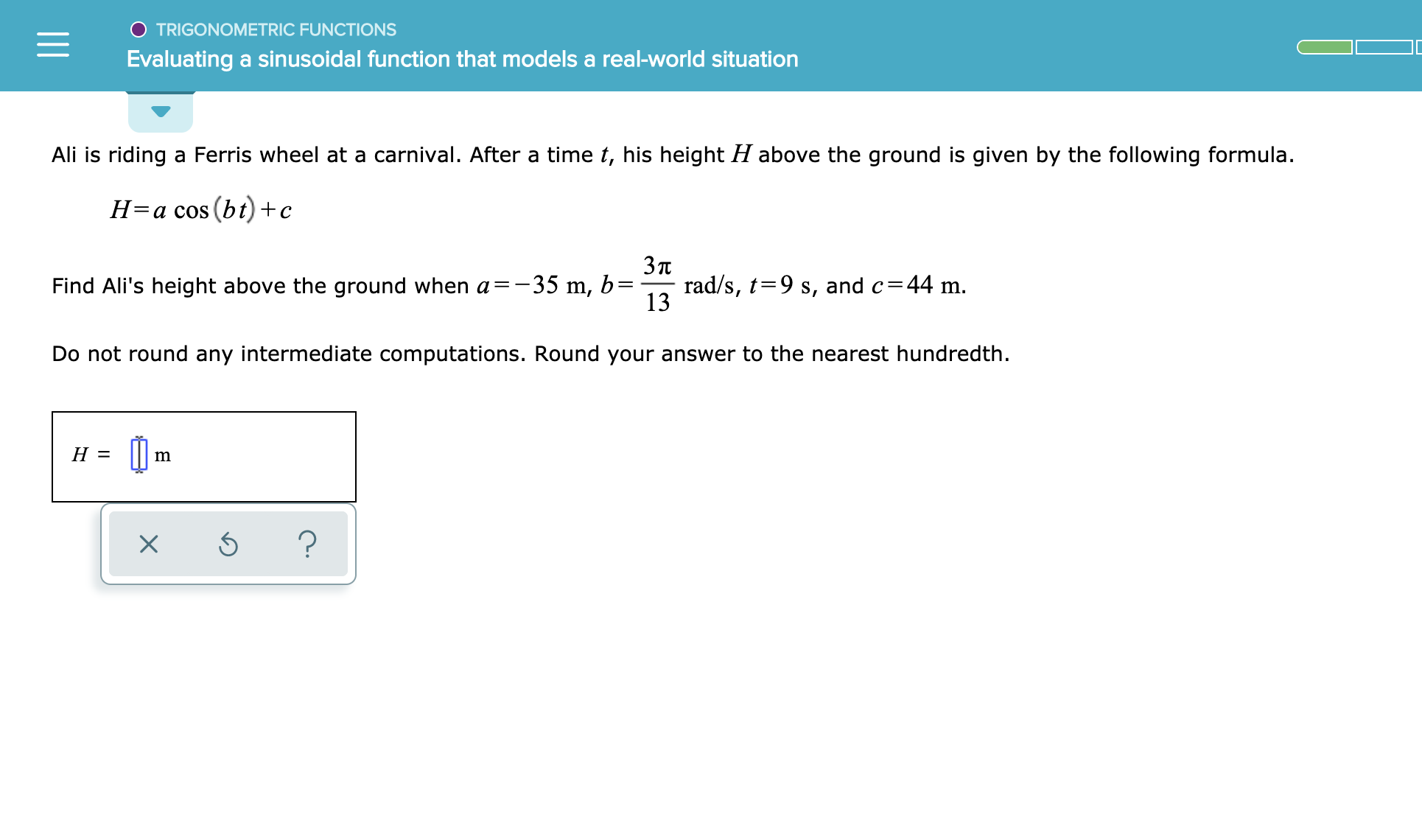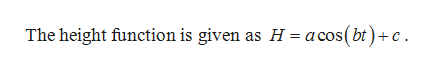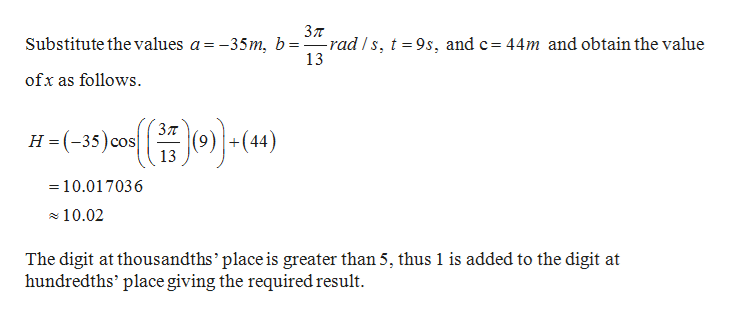TRIGONOMETRIC FUNCTIONSEvaluating a sinusoidal function that models a real-world situationAli is riding a Ferris wheel at a carnival. After a time t, his height H above the ground is given by the following formula(bt)+cН-а сos3пrad/s, t 9 s, and c 44 m.13Find Ali's height above the ground when a-35 m, b=Do not round any intermediate computations. Round your answer to the nearest hundredthН-?

Question

See attachedhelp_outlineImage TranscriptioncloseTRIGONOMETRIC FUNCTIONS Evaluating a sinusoidal function that models a real-world situation Ali is riding a Ferris wheel at a carnival. After a time t, his height H above the ground is given by the following formula (bt)+c Н-а сos 3п rad/s, t 9 s, and c 44 m. 13 Find Ali's height above the ground when a-35 m, b= Do not round any intermediate computations. Round your answer to the nearest hundredth Н- ? fullscreen
Step 1

Given:help_outlineImage TranscriptioncloseThe height function is given as H- acos(bt)+c fullscreen
Step 2

Calculatio...help_outlineImage TranscriptioncloseЗл Substitute the values a = -35m, b=-rad/s, t =9s, and c= 44m and obtain the value 13 ofx as follows H(-33)c (4) cos = = 10.017036 10.02 The digit at thousandths' place is greater than 5, thus 1 is added to the digit at hundredths' place giving the required result fullscreen

Want to see the full answer?

See Solution

Want to see this answer and more?

Our solutions are written by experts, many with advanced degrees, and available 24/7

See Solution
Tagged in

Functions